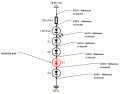# Diode voltage drops in a open circuit - Fundamental question

#### John Czerwinski

Joined Jun 19, 2017
62
I'm new to electronics and put together this simple circuit to practice measurement and troubleshooting. I understand in an open circuit, obviously there's no current flowing, but voltage is present. For non-active electronic components, there should be no voltage drop except for the failed component.

My question is for the circuit below: Although the circuit becomes open because of the reversed diode bias at D3, there's still voltage drops on the LED, D1, and D2. Is this because, there's still a Vf of semiconductors (diffusion region) that still cause a voltage drop? I've seen this on transistors used as a switch as well (NPN collector when the base has no current following and the transistor is in the cut-off region).#### AlbertHall

Joined Jun 4, 2014
12,293
There will still be leakage current through D3 and the voltmeter will draw current from the circuit.
Try connecting the meter directly across D1, D2 and the LED.

#### OBW0549

Joined Mar 2, 2015
3,566
Although the circuit becomes open because of the reversed diode bias at D3, there's still voltage drops on the LED, D1, and D2. Is this because, there's still a Vf of semiconductors (diffusion region) that still cause a voltage drop?
No, it's because you're mistaken in your belief that there's no current flowing: your meter draws current because of its finite input resistance (usually 10 MΩ), and it is this current which is causing the voltage drops you're observing.

#### John Czerwinski

Joined Jun 19, 2017
62
Thank you for the quick responses. Again, I'm new and just trying to get understanding...

If I take D3 out of the circuit, there wouldn't be any leaky circuit, but I still get the same results.
If the meter is drawing circuit, because of its inherent resistance, shouldn't there be some voltage drop on the 100 ohm resistor?

#### AlbertHall

Joined Jun 4, 2014
12,293
If the meter is drawing circuit, because of its inherent resistance, shouldn't there be some voltage drop on the 100 ohm resistor?
Ther will be but the current is very low so the voltage drop will be very low, below wht your meter can measure.

•John Czerwinski

#### OBW0549

Joined Mar 2, 2015
3,566
If the meter is drawing circuit, because of its inherent resistance, shouldn't there be some voltage drop on the 100 ohm resistor?
Of course there will be. The voltage drop will be approximately 8.95V * 100Ω / 10 MΩ, or 89.5 μV. And unless you've got at least a 6-digit DVM, you're not going to see it.

•John Czerwinski

#### John Czerwinski

Joined Jun 19, 2017
62
Of course there will be. The voltage drop will be approximately 8.95V * 100Ω / 10 MΩ, or 89.5 μV. And unless you've got at least a 6-digit DVM, you're not going to see it.
I have another meter and measured the current of my first meter (the one I used to build the diagram). There's .06 uA. So, the voltage drop of the resistor is too small for either of my meters to measure.

Last question, your formula is 8.95V * 100Ω / 10 MΩ, or 89.5 μV where did you come up with that? Could I use:

V drop = I (meter) x R (parallel of 100 resistor and 10M for the meter)
V drop = .00000006 x (100 || 10,000,000)
.00000594 = .00000006 x 99

#### OBW0549

Joined Mar 2, 2015
3,566
Last question, your formula is 8.95V * 100Ω / 10 MΩ, or 89.5 μV where did you come up with that? Could I use:

V drop = I (meter) x R (parallel of 100 resistor and 10M for the meter)
V drop = .00000006 x (100 || 10,000,000)
.00000594 = .00000006 x 99
Nope. The 100 ohm and your meter's resistance are in series, not parallel. (Note that I am NOT referring to a meter being used to measure the voltage drop across the 100 ohm resistor, but rather to the meter being used to measure the voltages at various points in the circuit with respect to ground-- that is, the one causing the current flow that you weren't initially aware of.)